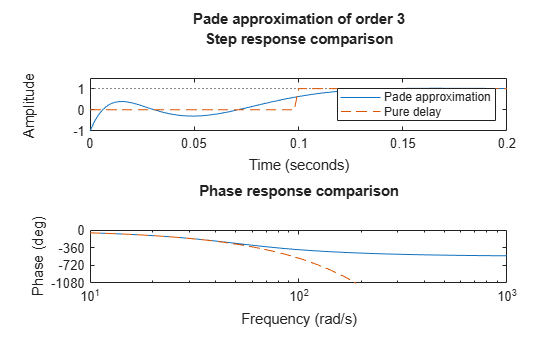Padé approximation of model with time delays

## Syntax

```[num,den] = pade(T,N) pade(T,N) sysx = pade(sys,N) sysx = pade(sys,NU,NY,NINT) ```

## Description

`pade` approximates time delays by rational models. Such approximations are useful to model time delay effects such as transport and computation delays within the context of continuous-time systems. The Laplace transform of a time delay of T seconds is exp(–sT). This exponential transfer function is approximated by a rational transfer function using Padé approximation formulas .

`[num,den] = pade(T,N) ` returns the Padé approximation of order `N` of the continuous-time I/O delay exp(–sT) in transfer function form. The row vectors `num` and `den` contain the numerator and denominator coefficients in descending powers of s. Both are `N`th-order polynomials.

When invoked without output arguments, `pade(T,N)` plots the step and phase responses of the `N`th-order Padé approximation and compares them with the exact responses of the model with I/O delay `T`. Note that the Padé approximation has unit gain at all frequencies.

`sysx = pade(sys,N) ` produces a delay-free approximation `sysx` of the continuous delay system `sys`. All delays are replaced by their `N`th-order Padé approximation. See Time Delays in Linear Systems for more information about models with time delays.

`sysx = pade(sys,NU,NY,NINT)` specifies independent approximation orders for each input, output, and I/O or internal delay. Here `NU`, `NY`, and `NINT` are integer arrays such that

• `NU` is the vector of approximation orders for the input channel

• `NY` is the vector of approximation orders for the output channel

• `NINT` is the approximation order for I/O delays (TF or ZPK models) or internal delays (state-space models)

You can use scalar values for `NU`, `NY`, or `NINT` to specify a uniform approximation order. You can also set some entries of `NU`, `NY`, or `NINT` to `Inf` to prevent approximation of the corresponding delays.

## Examples

collapse all

Compute a third-order Padé approximation of a 0.1-second I/O delay.

```s = tf('s'); sys = exp(-0.1*s); sysx = pade(sys,3)```
```sysx = -s^3 + 120 s^2 - 6000 s + 1.2e05 -------------------------------- s^3 + 120 s^2 + 6000 s + 1.2e05 Continuous-time transfer function. ```

Here, `sys` is a dynamic system representation of the exact time delay of 0.l s. `sysx` is a transfer function that approximates that delay.

Compare the time and frequency responses of the true delay and its approximation. Calling the `pade` command without output arguments generates the comparison plots. In this case the first argument to `pade` is just the magnitude of the exact time delay, rather than a dynamic system representing the time delay.

`pade(0.1,3)`## Limitations

High-order Padé approximations produce transfer functions with clustered poles. Because such pole configurations tend to be very sensitive to perturbations, Padé approximations with order `N>10` should be avoided.

## References

 Golub, G. H. and C. F. Van Loan, Matrix Computations, Johns Hopkins University Press, Baltimore, 1989, pp. 557-558.